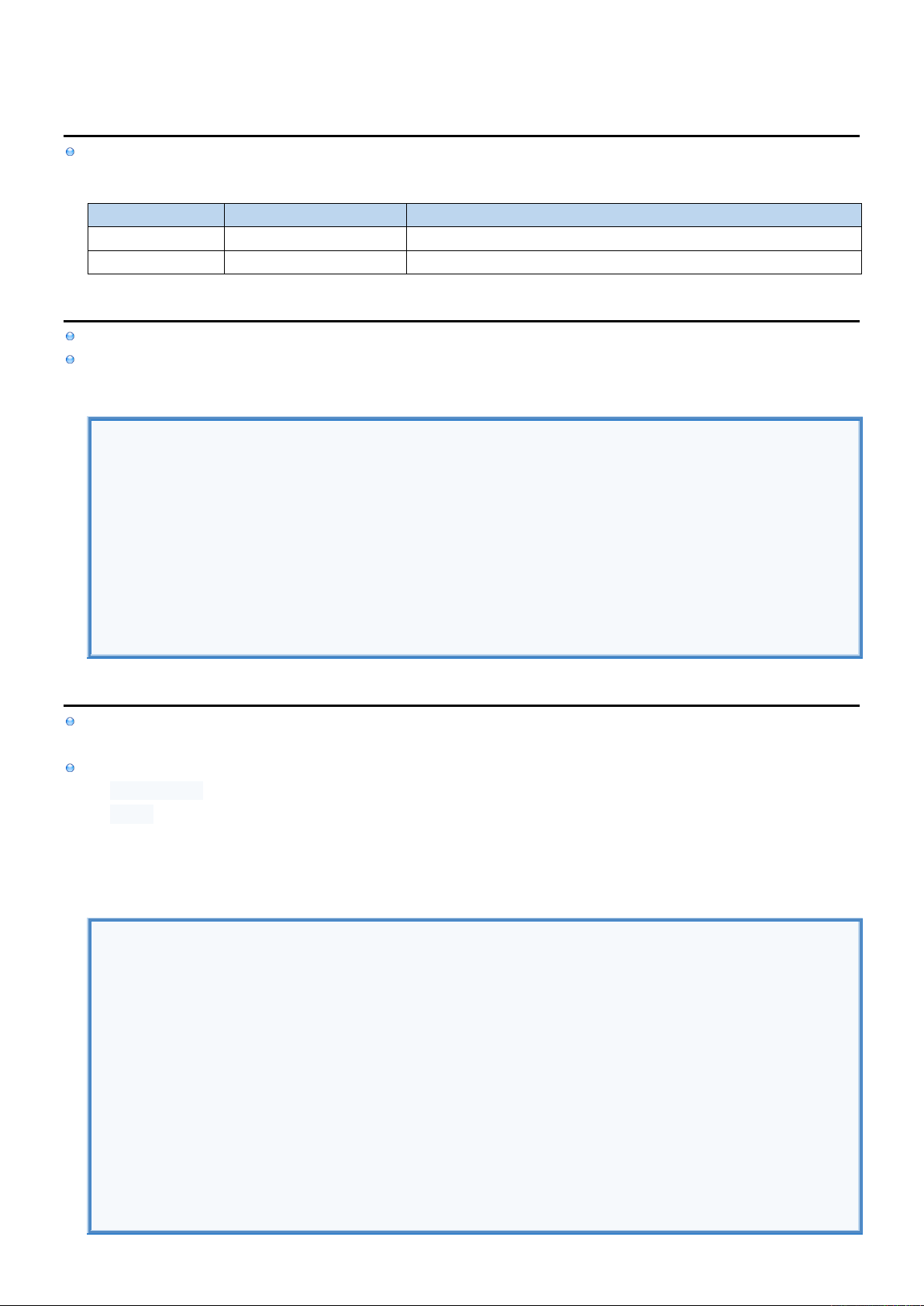2
2
.
.
1
1
.
.
3
3
C
C
o
o
l
l
l
l
e
e
c
c
t
t
i
i
o
o
n
n
s
s
I
I
n
n
f
f
o
o
Collection Data Types are implemented as Structures and allow us to group multiple items of the same Data Type.
Collection Data Types
TYPE
EXAMPLE
DESCRIPTION
String
Class for storing constant strings.
Array
Data of the same Data Type.
S
S
t
t
r
r
i
i
n
n
g
g
String Data Type represents Array of Characters and can be created using String Literal.
Check out String cheat sheet containing code samples for working with String data type.
String Data Type
//DECLARE STRINGS.
var name : String = "John" //Explicit Data Type declaration.
var name1 = "John" //Implicit Data Type declaration.
//ACESS CHARACTERS.
var length = name.length // 4
var firstCharInName = name // 'J'
var lastCharInName = name[length - 1] // 'n'
//CONCATENATE & REFERENCE VARIABLES.
var age : Int = 20
print(name + " is " + "\$age years old.")
A
A
r
r
r
r
a
a
y
y
[
[
R
R
]
]
[
[
R
R
]
]
In Kotlin Arrays are represented using Array<T> class. (Array<Int> is translated to Integer[])
There is also IntArray(1,2,3) which is translated as int[] and can be converted using IntArray.toTypedArray().
Array can be created using
arrayOf<Int> () library Function
Array() Constructor
first parameter is the size of Array
second parameter is function that takes array index and returns element to be inserted at that index
Array
//DECLARE ARRAYS USING: arrayOf() library function
var integers1 : Array<Int> = arrayOf<Int>(1, 2, 3, 4, 5)
var integers2 : Array<Int> = arrayOf (1, 2, 3, 4, 5)
var integers3 = arrayOf<Int>(1, 2, 3, 4, 5)
var integers = arrayOf (1, 2, 3, 4, 5)
var strings = arrayOf ("Cat", "Dog", "Lion", "Tiger")
var objects = arrayOf (1, true, 3, "Hello", 'A') //Mixed Data Types
//DECLARE ARRAYS USING: Array() Constructor
var mySquareArray = Array(5, {i -> i * i}) // [0, 1, 4, 9, 16]
//ACCESS ELEMENTS.
val size = integers.size
val first = integers
val last = integers[size - 1]
integers = 10